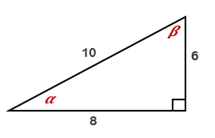Chapter 11.CT, Problem 2CTElementary Geometry For College St...

7th Edition
Alexander + 2 others
ISBN: 9781337614085

Solutions

Chapter
SectionElementary Geometry For College St...

7th Edition
Alexander + 2 others
ISBN: 9781337614085
Textbook Problem

For the right triangle shown, express each ratio as a fraction in lowest terms:a) cos βb) sin α

To determine

a

To express:

The ratio cos β in the lowest term.

Explanation

Definition:

In a right triangle, the cosine ratio for an acute angle is the ratio adjacent sidehypotenuse.

Given:

The right triangle shown below

Calculation:

From the figure given,

For the angle β, adjacent side = 6 and hypotenuse = 10

To determine

b

To express:

The given sin α in the lowest term.

Still sussing out bartleby?

Check out a sample textbook solution.

See a sample solution

The Solution to Your Study Problems

Bartleby provides explanations to thousands of textbook problems written by our experts, many with advanced degrees!

Get Started

Find more solutions based on key concepts